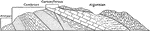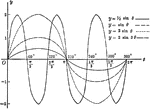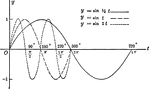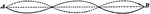Rock Layers Showing Geological Periods

"Diagrammatic structure section of the Wasatch Range in Ogden Canyon." -Lee, 1915Sine Curves y= sin Ǝ, y= 1/2 sin Ǝ, y=2 sin Ǝ, y= 2 sin 3Ǝ

Sine curves of varying frequency and amplitude plotted from 0 to 2 pi. Graph of y= sin θ, y= 1/2…Sine Curves y= sin t, y= r sin1/2t, y= r sin 2t

Sine curves of varying frequency plotted from 0 to 2 pi. Graph of y= sin t, y= r sin1/2t, y=r sin 2t.Waves with Equal Periods and Opposite Amplitudes

"...represents two wave systems of equal periods and amplitudes but of opposite phases." -Avery 1895